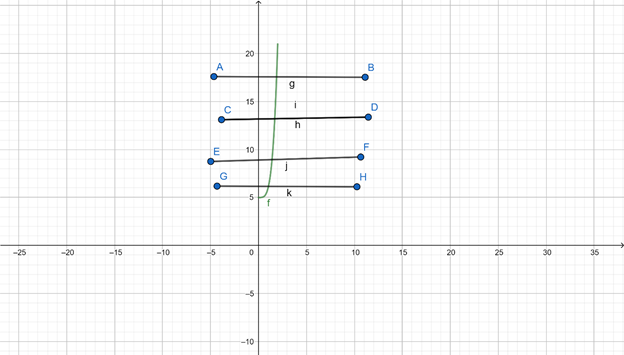# To check if the function f ( x ) = x 4 + 5 is one to one.### Precalculus: Mathematics for Calcu...

6th Edition
Stewart + 5 others
Publisher: Cengage Learning
ISBN: 9780840068071### Precalculus: Mathematics for Calcu...

6th Edition
Stewart + 5 others
Publisher: Cengage Learning
ISBN: 9780840068071

#### Solutions

Chapter 2.7, Problem 18E
To determine

## To check if the function f(x)=x4+5 is one to one.

Expert Solution

The function f(x)=x4+5 one to one.

### Explanation of Solution

Given is the function f(x)=x4+5 and 0x2

Concept used: To check if a function if one to one. Horizontal line test can be used.

If the line cuts graph at only one point , then the function is one to one.

GraphInterpretation: from the above graph, The graph starts from the coordinate (0,5) because the value of x is between 0 to 2. And the horizontal lines cut the graph at only one point. So that means the function f(x)=x4+5 is one to one.

### Have a homework question?

Subscribe to bartleby learn! Ask subject matter experts 30 homework questions each month. Plus, you’ll have access to millions of step-by-step textbook answers!